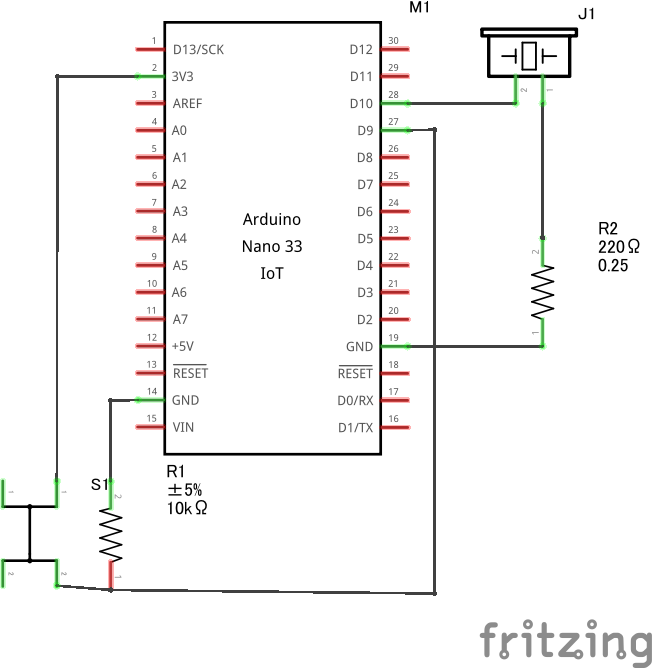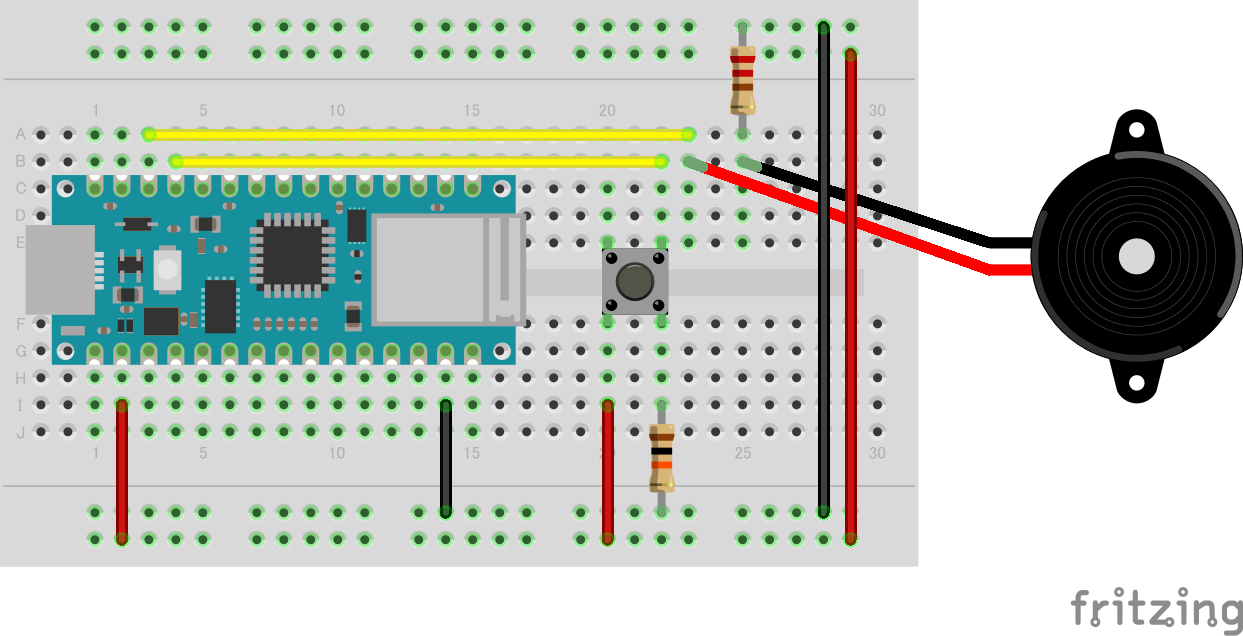# Week 2: Why Won't This Speaker Work?! Because I Need Delay!

Idea: Create an instrument using 8 push buttons corresponding to each note in a scale, a speaker, and the Arduino to assign different frequency pitches when each button is pressed. As an extension, change the key of the instrument by turning a potentiometer up or down.

Reality: My speaker won’t even play one note! What is wrong with my circuit?An Arduino connected to a push button, which in turn connects to both a 10kΩ resistor that leads to ground as well as D9. Another wire connects D10 to a speaker, which follows a 220Ω resistor to ground.A breadboard visualization of the circuit described above.

I wrote the following code for this section of the circuit:

``````const int outputPin = 10;
int frequency = 850;

void setup() {
pinMode(9, INPUT);
pinMode(outputPin, OUTPUT);
}

void loop() {
tone(outputPin, frequency);
} else {
noTone(outputPin);
}
}
``````

As a check, I replaced the speaker with a red LED, and changed the code so that instead of the tone() and noTone() functions, I used digitalWrite(). The circuit works as expected:

Why doesn’t the circuit work with a speaker? After trying out a few things with Elias, I found out that adding a few milliseconds of delay will do the trick. But why do we need delay to produce sound?

``````if (digitalRead(9) == HIGH) {
tone(outputPin, 523);
delay(10);
}
``````

Anyway, since my instrument is basically a group of buttons, I can repeat the same logic above for the rest. Is it possible to simplify the circuit? For example, I currently have a 10kΩ resistor connected to each push button, but if I connect all of the ends, can I use just one?

And how much more can I simplify the code? If there are arithmetic relationships between each note in a scale, I can just calculate it, allowing the user to potentially tune the instrument to whatever key they like.

Complete code:

``````const int speakerPin = 10;
int baseFrequency = 523;
int scale = {523, 587, 659, 698, 784, 880, 988, 1047}; // C5 Major scale

void setup() {
pinMode(9, INPUT);
pinMode(8, INPUT);
pinMode(7, INPUT);
pinMode(6, INPUT);
pinMode(5, INPUT);
pinMode(4, INPUT);
pinMode(3, INPUT);
pinMode(2, INPUT);
pinMode(speakerPin, OUTPUT);
}

void loop() {
tone(speakerPin, scale);
} else if (digitalRead(8) == HIGH) {
tone(speakerPin, scale);
} else if (digitalRead(7) == HIGH) {
tone(speakerPin, scale);
} else if (digitalRead(6) == HIGH) {
tone(speakerPin, scale);
} else if (digitalRead(5) == HIGH) {
tone(speakerPin, scale);
} else if (digitalRead(4) == HIGH) {
tone(speakerPin, scale);
} else if (digitalRead(3) == HIGH) {
tone(speakerPin, scale);
} else if (digitalRead(2) == HIGH) {
tone(speakerPin, scale);
}
else {
noTone(speakerPin);
}
delay(10);
}
``````